# Instant Centers for a CrankSlider Introduction Velocity Analysis

• Slides: 6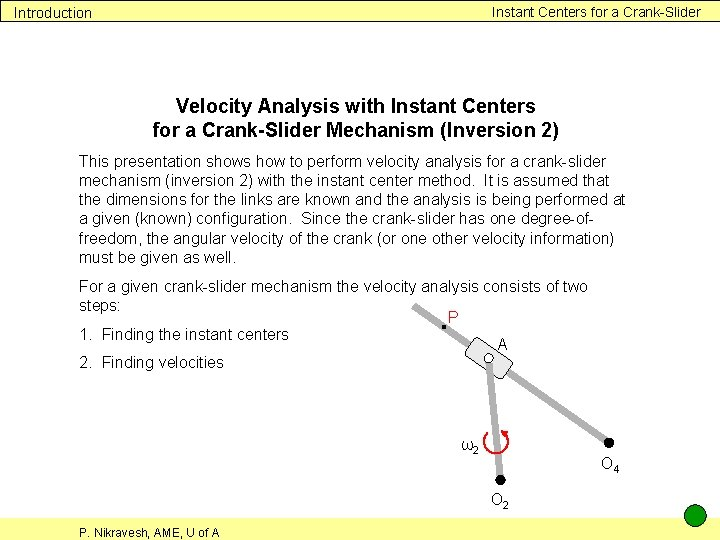Instant Centers for a Crank-Slider Introduction Velocity Analysis with Instant Centers for a Crank-Slider Mechanism (Inversion 2) This presentation shows how to perform velocity analysis for a crank-slider mechanism (inversion 2) with the instant center method. It is assumed that the dimensions for the links are known and the analysis is being performed at a given (known) configuration. Since the crank-slider has one degree-offreedom, the angular velocity of the crank (or one other velocity information) must be given as well. For a given crank-slider mechanism the velocity analysis consists of two steps: P 1. Finding the instant centers A 2. Finding velocities ω2 O 4 O 2 P. Nikravesh, AME, U of A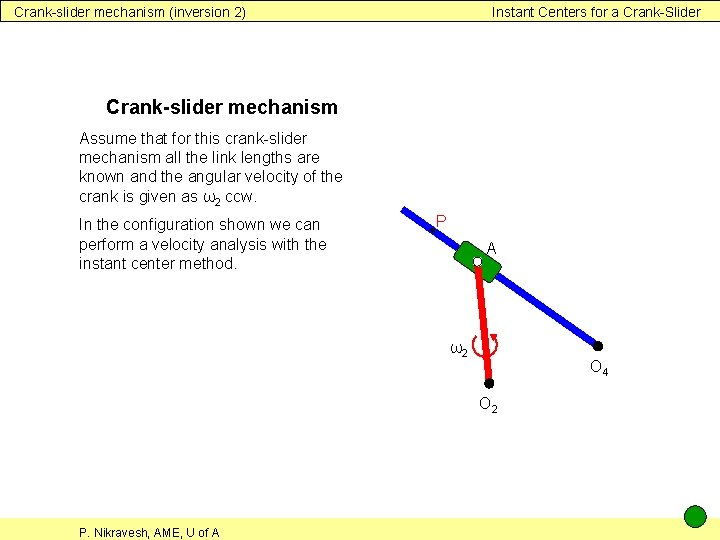Crank-slider mechanism (inversion 2) Instant Centers for a Crank-Slider Crank-slider mechanism Assume that for this crank-slider mechanism all the link lengths are known and the angular velocity of the crank is given as ω2 ccw. In the configuration shown we can perform a velocity analysis with the instant center method. P A ω2 O 4 O 2 P. Nikravesh, AME, U of A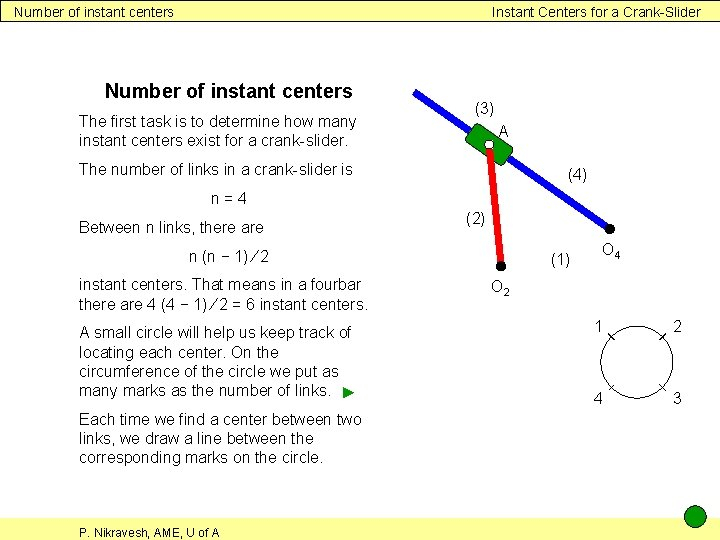Number of instant centers Instant Centers for a Crank-Slider Number of instant centers The first task is to determine how many instant centers exist for a crank-slider. (3) A The number of links in a crank-slider is (4) n=4 Between n links, there are (2) n (n − 1) ∕ 2 instant centers. That means in a fourbar there are 4 (4 − 1) ∕ 2 = 6 instant centers. A small circle will help us keep track of locating each center. On the circumference of the circle we put as many marks as the number of links. ► Each time we find a center between two links, we draw a line between the corresponding marks on the circle. P. Nikravesh, AME, U of A (1) O 4 O 2 1 2 4 3Finding the instant centers Instant Centers for a Crank-Slider Finding the instant centers Four of the centers are already known: They are three pin joints and the sliding joint. ► (3) Note: The instant center between two bodies connected by a slider is located in infinity on any axis perpendicular to the axis of sliding. We don’t know I 1, 3 but we know that it lies on the same line as I 4, 1 and I 3, 4. ► I 1, 3 also lies on the same line as I 1, 2 and I 2, 3. ► The point of intersection is I 1, 3. ► I 2, 4 is also unknown but it lies on the same line as I 3, 4 and I 2, 3. ► I 2, 4 also lies on the same line as I 4, 1 and I 1, 2. ► The point of intersection is I 2, 4. ► Now we have found all 6 centers. P. Nikravesh, AME, U of A = I 3, 4 A = I 2, 3 (4) (2) O 4 = I 4, 1 (1) O 2 = I 1, 2 I 2, 4 1 2 4 3 I 1, 3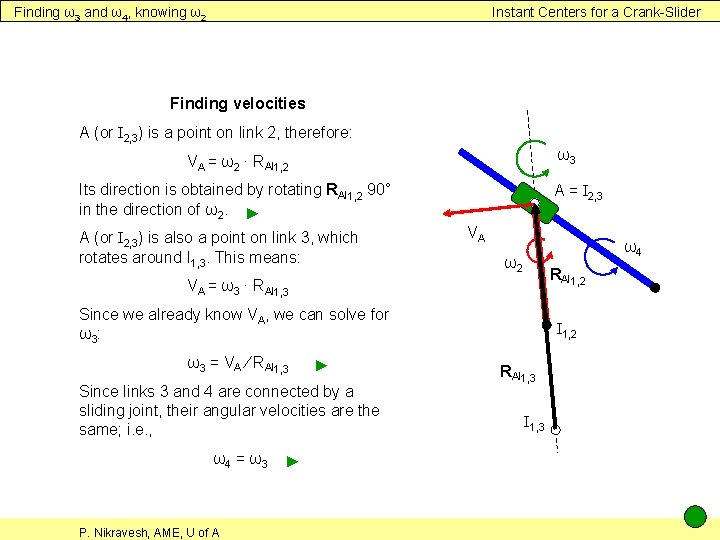Finding ω3 and ω4, knowing ω2 Instant Centers for a Crank-Slider Finding velocities A (or I 2, 3) is a point on link 2, therefore: ω3 VA = ω2 ∙ RAI 1, 2 Its direction is obtained by rotating RAI 1, 2 90° in the direction of ω2. ► A (or I 2, 3) is also a point on link 3, which rotates around I 1, 3. This means: A = I 2, 3 VA ω4 ω2 RAI 1, 2 VA = ω3 ∙ RAI 1, 3 Since we already know VA, we can solve for ω 3: ω3 = VA ∕ RAI 1, 3 ► Since links 3 and 4 are connected by a sliding joint, their angular velocities are the same; i. e. , ω4 = ω3 P. Nikravesh, AME, U of A ► I 1, 2 RAI 1, 3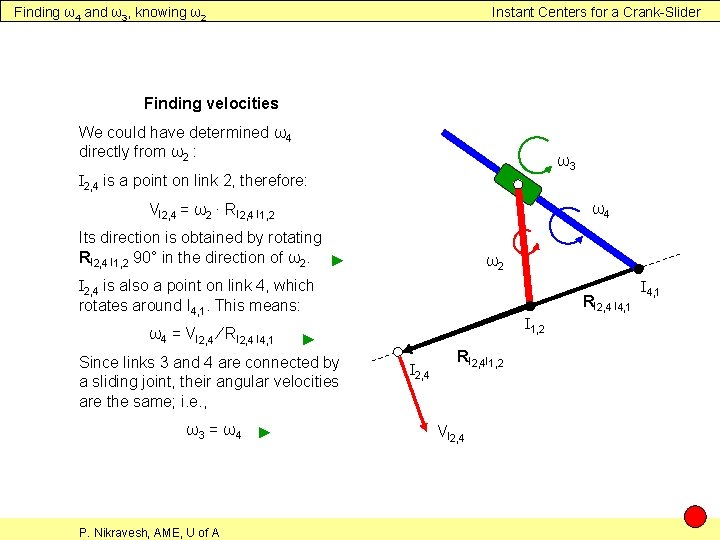Finding ω4 and ω3, knowing ω2 Instant Centers for a Crank-Slider Finding velocities We could have determined ω4 directly from ω2 : ω3 I 2, 4 is a point on link 2, therefore: ω4 VI 2, 4 = ω2 ∙ RI 2, 4 I 1, 2 Its direction is obtained by rotating RI 2, 4 I 1, 2 90° in the direction of ω2. ► ω2 I 2, 4 is also a point on link 4, which rotates around I 4, 1. This means: RI 2, 4 I 4, 1 I 1, 2 ω4 = VI 2, 4 ∕ RI 2, 4 I 4, 1 ► Since links 3 and 4 are connected by a sliding joint, their angular velocities are the same; i. e. , ω3 = ω4 P. Nikravesh, AME, U of A ► I 2, 4 RI 2, 4 I 1, 2 VI 2, 4 I 4, 1